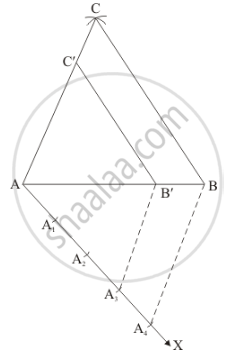# Draw a δAbc In Which Bc = 6 Cm, Ab = 4 Cm And Ac = 5 Cm. Draw a Triangle Similar to δAbc With Its Sides Equal to (3/4)Th Of the Corresponding Sides of δAbc. - Mathematics

Draw a ΔABC in which BC = 6 cm, AB = 4 cm and AC = 5 cm. Draw a triangle similar to ΔABC with its sides equal to (3/4)th of the corresponding sides of ΔABC.

#### Solution

Given that

Construct a triangle of sides BC = 6 cm, AB = 4 cm and AC = 5 cm and then a triangle similar to it whose sides are (3/4)th of the corresponding sides of ΔABC.

We follow the following steps to construct the givenStep of construction

Step: I- First of all we draw a line segment AB = 4 cm.

Step: II- With as centre and radius = AC = 5 cm, draw an arc.

Step: III -With as centre and radius = BC = 6 cm, draw an arc, intersecting the arc drawn in step II at C.

Step: IV -Joins AC and BC to obtain ΔABC.

Step: V -Below AB, makes an acute angle ∠BAX = 60°.

Step: VI -Along AX, mark off four points A1, A2, A3 and A4 such that AA1 = A1A2 = A2A3 = A3A4

Step: VII -Join A4B.

Step: VIII -Since we have to construct a triangle each of whose sides is (3/4)th of the corresponding sides of ΔABC.

So, we take three parts out of four equal parts on AX from point A3 draw A3B' || A4B, and meeting AB at B’.

Step: IX- From B’ draw B'C || BC and meeting AC at C’

Thus, ΔAB'C' is the required triangle, each of whose sides is (3/4)th of the corresponding sides of ΔABC.

Concept: Division of a Line Segment
Is there an error in this question or solution?

#### APPEARS IN

RD Sharma Class 10 Maths
Chapter 9 Constructions
Exercise 9.2 | Q 4 | Page 9